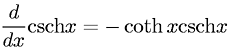Equations > Calculus > Differentiation/Differentals > Derivative of Hyperbolic Cosecant

### Derivative of Hyperbolic CosecantLatex Code:

MathML Code:

 $\fracd\mathrm{dx}\mathrm{csch}\nolimitsx=-\mathrm{coth}x\mathrm{csch}\nolimitsx$

MathType 5.0: